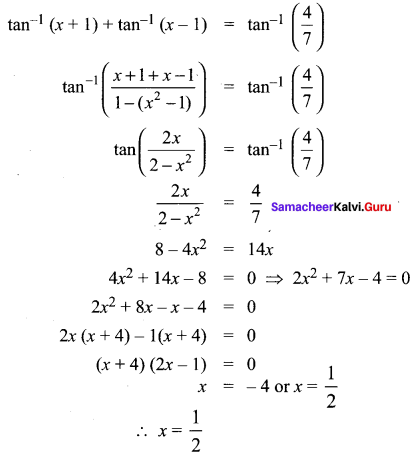## Tamilnadu Samacheer Kalvi 11th Maths Solutions Chapter 3 Trigonometry Ex 3.11

Question 1.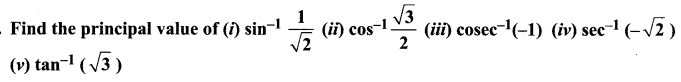Solution: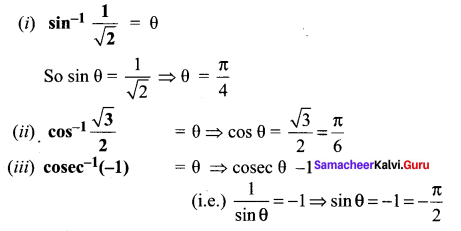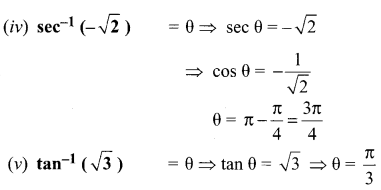Question 2.
A man standing directly opposite to one side of a road of width x meter views a circular shaped traffic green signal of diameter a meter on the other side of the road. The bottom of the green signal is b meter height from the horizontal level of viewer’s eye. If a denotes the angle subtended by the diameter of the green signal at the viewer’s eye, then prove that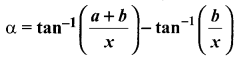Solution: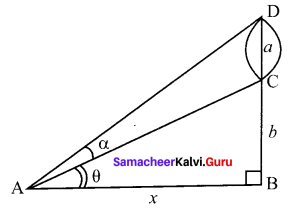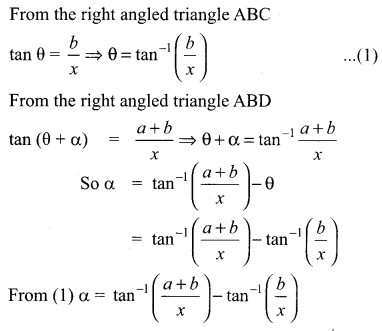### Samacheer Kalvi 11th Maths Solutions Chapter 3 Trigonometry Ex 3.11 Additional Questions

Question 1.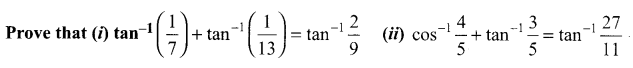Solution: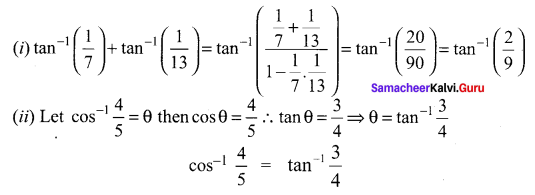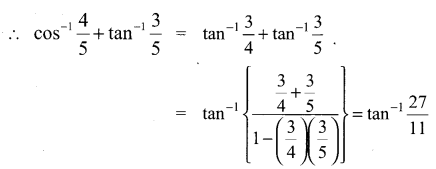Question 2.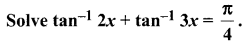Solution: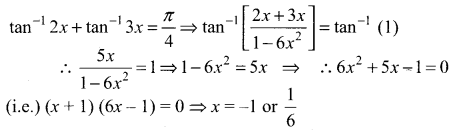The negative value of x is rejected since it makes RHS negative
∴ x = $$\frac{1}{6}$$

Question 3.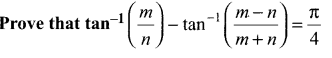Solution: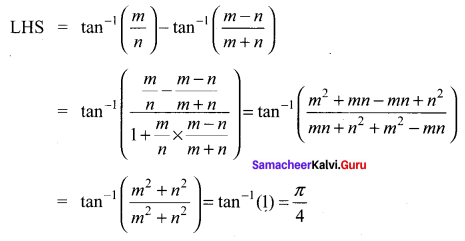Question 4.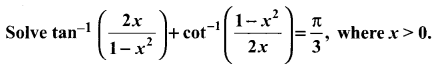Solution: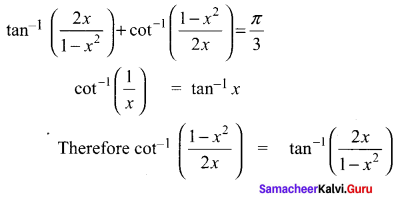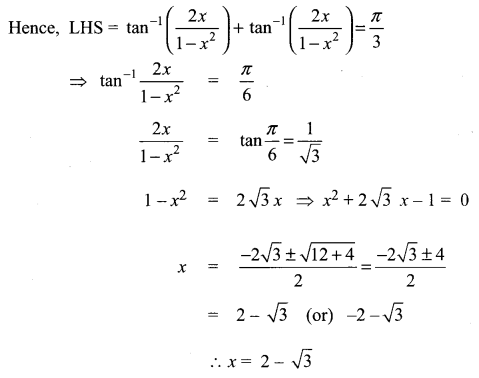Question 5.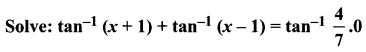Solution: